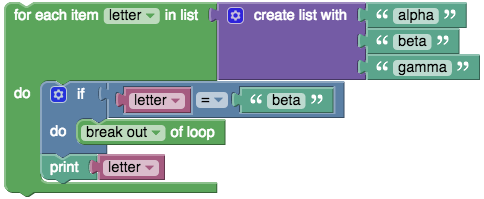en
uk

# LOOPS

A loop statement allows users to execute a statement or group of statements multiple times. It can also be seen as a way to repeat a task more than one time.

Simple Repeat block

The simplest "repeat" block runs the code in its body the specified number of times. For example, the following block will print "Hello world!" 10 times.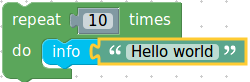Repeat While
Repeats a statement or group of statements while a given condition is True. If the condition is false, then the loop will end.  For example, Imagine a game in which a player rolls a die and adds up all of the values rolled as long as the total is less than 30. The following blocks implement that game:

1. A variable named total gets an initial value of 0.
2. The loop begins with a check that total is less than 30. If so, the blocks in the body are run.
3. A random number in the range 1 to 6 is generated (simulating a die roll) and stored in a variable named roll.
4. The number rolled is printed.
5. The variable total gets increased by roll.
6. The end of the loop having been reached, control goes back to step 2.

When the loop completes, any subsequent blocks (not shown) would be run. In our example, the loop would end after some number of random numbers in the range 1 to 6 had been printed, and the variable total would hold the sum of these numbers, which would be guaranteed to be at least 30.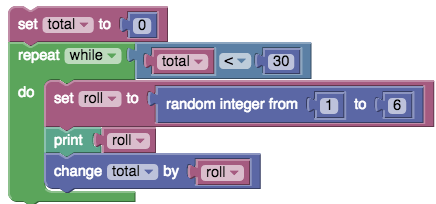Repeat Until loop
This is similar to the While loop except that it repeats the statement or group of statements until the condition is true. Here, the loop is contained until the sum of the rolled numbers is greater than or equal to 30.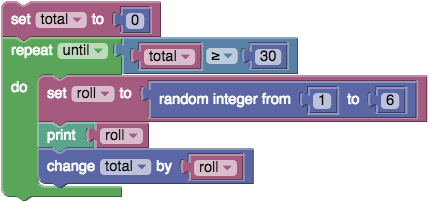For each loop
For each block is designed for data structures which can either be an array or lists. They are designed along the format that for each object in the data structure, a certain action is performed. For the example below, the program prints each element of the list: "alpha", "beta", "gamma".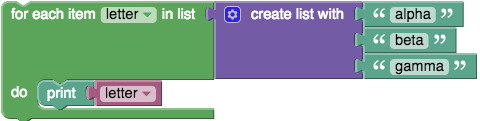Continue with next iteration loop
This block causes the program to skip the remaining code in body to the next iteration of the loop. The following program prints "alpha" on the first iteration of the loop. On the second iteration, the continue with next iteration block is run, skipping the printing of "beta". On the final iteration, "gamma" is printed.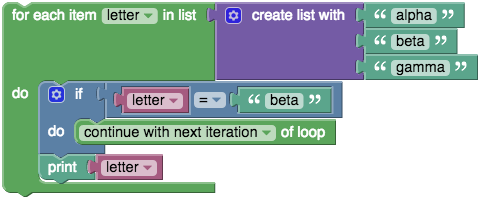Note: The Repeat While and Until loops are on the same block. To use either one of them drag and drop the Repeat While block to the programming space then click on the downward arrow beside While. You will now see a dropdown list where you can select either While or Until. The Break out or Continue with next iteration block is also used in the same way.

Break out of loop
The break out of loop block provides an early exit from a loop. The following program prints "alpha" on the first iteration and "breaks out" of the loop on the second iteration when the loop variable is equal to "beta". The "gamma" item in the list is never reached.